## 队列

### 如何理解队列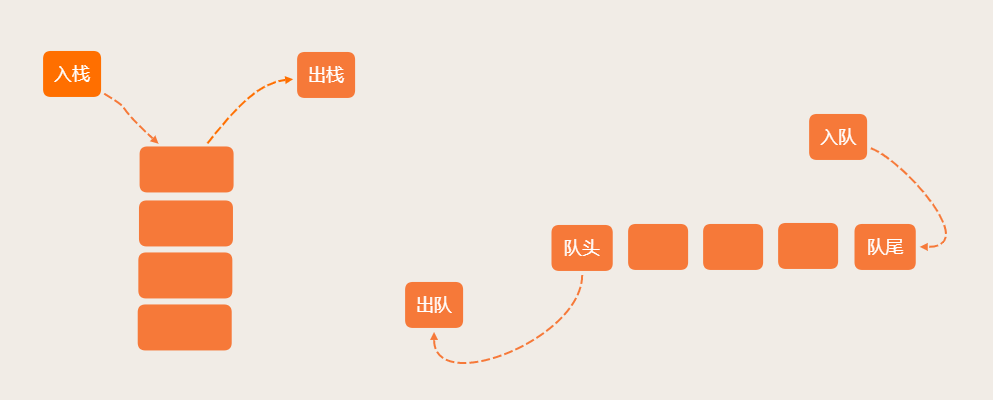### 顺序队列和链式队列

``````class ArrayQueue {
items = null;
n = 0;
tail = 0;

constructor (capacity) {
this.items = new Array(capacity);
this.n = capacity;
}

enqueue (item) {
if (this.tail === this.n) return false;

this.items[this.tail] = item;
this.tail++;

return true;
}

dequeue () {
if (this.head === this.tail) return null;

return ret;
}
}
``````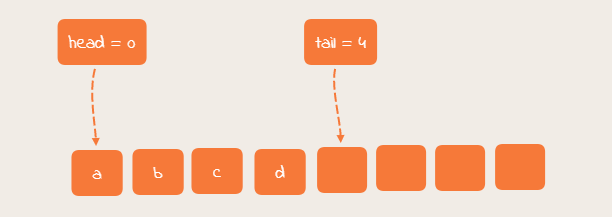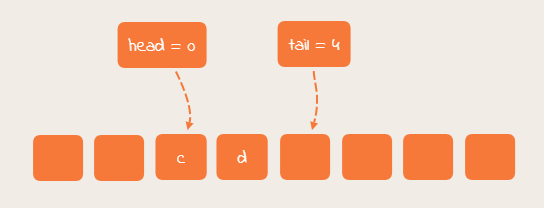``````class ArrayQueue {
items = null;
n = 0;
tail = 0;

constructor (capacity) {
this.items = new Array(capacity);
this.n = capacity;
}

enqueue (item) {
if (this.tail === this.n) {
if (this.head === 0) return false;

for (let i = this.head; i < this.tail; i++) {
this.items[ i - this.head] = this.items[i];
}

};

this.items[this.tail] = item;
this.tail++;

return true;
}

dequeue () {
if (this.head === this.tail) return null;

return ret;
}
}
``````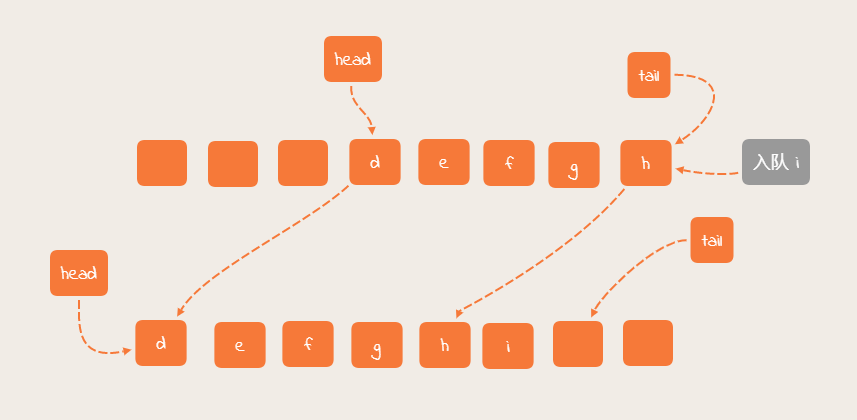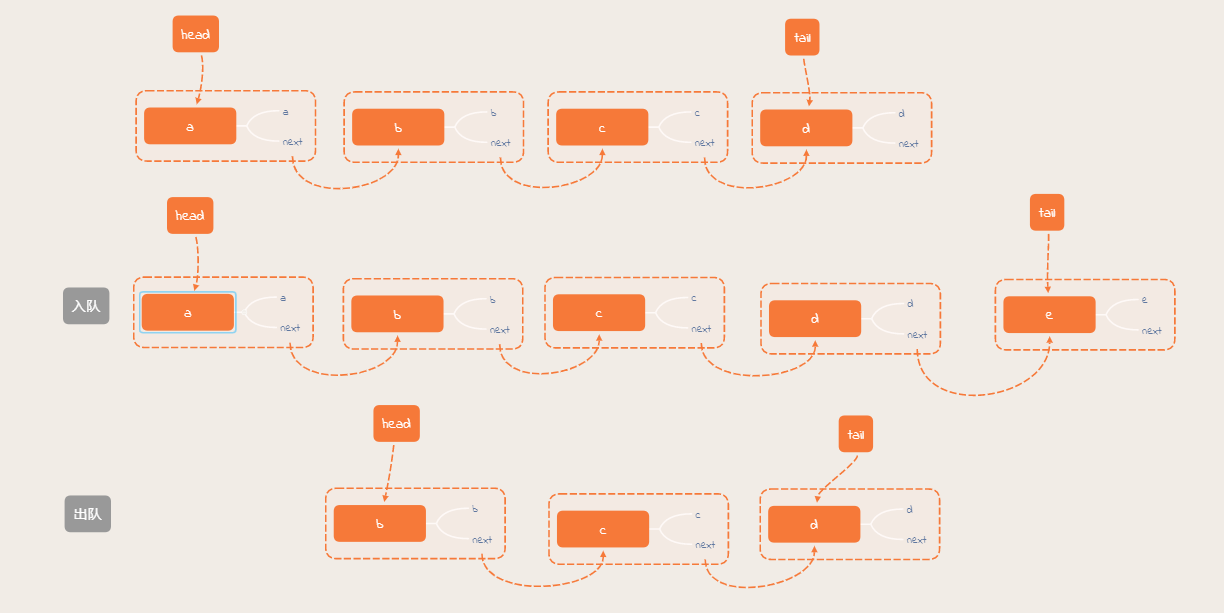### 循环队列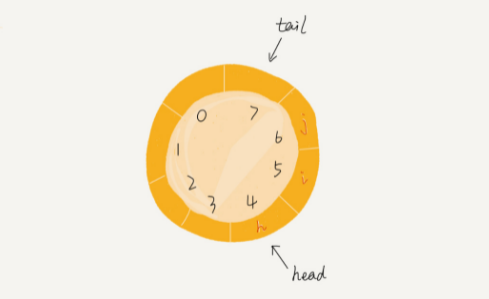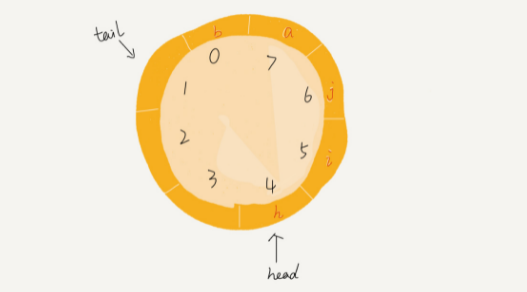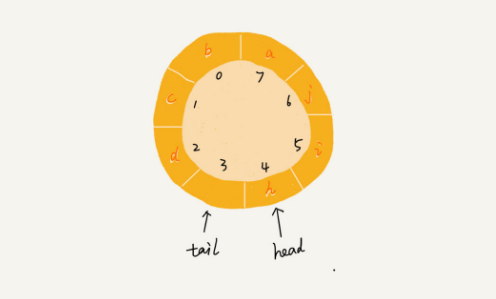``````class CircularQueue {
items = null;
n = 0;
tail = 0;

constructor (capacity) {
this.items = new Array(capacity);
this.n = capacity;
}

enqueue (item) {
if ((this.tail + 1) % this.n === this.head) return false;

this.items[this.tail] = item;
this.tail = (this.tail + 1) % this.n;

return true;
}

dequeue () {
if (this.head === this.tail) return null;

return ret;
}
}
``````

### 循环队列和并发队列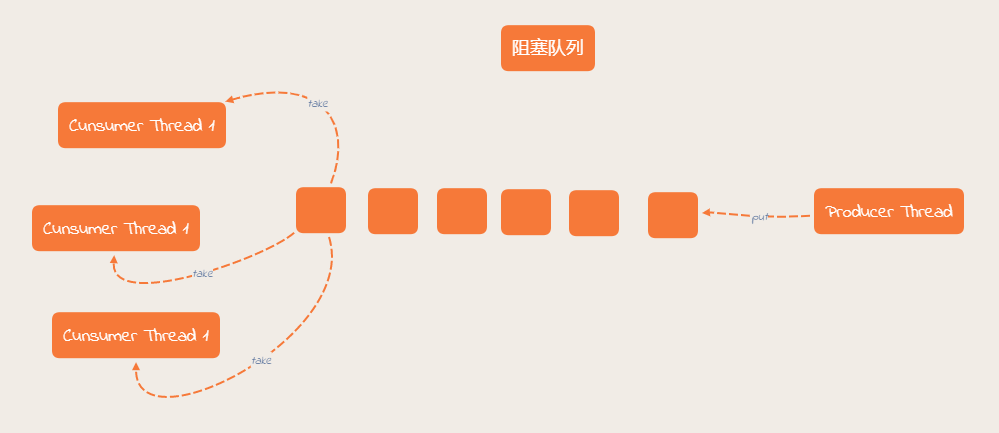### 技术拓展

#### 队列排队请求的应用场景

• 分布式锁中的消息队列 kafka
• sockets 网络连接队列
• 数据库连接队列

#### 如何实现无锁并发队列

CAS 实现无锁队列。

CAS（Compare and Swap），比较再交换。

CAS 是一种无锁算法，CAS 有 3 个操作数，内存值 V，旧的预期值 A，要修改的新值 B。当且仅当预期值 A 和 内存值 V 相同时，将内存值 V 修改为 B，否则什么都不做。